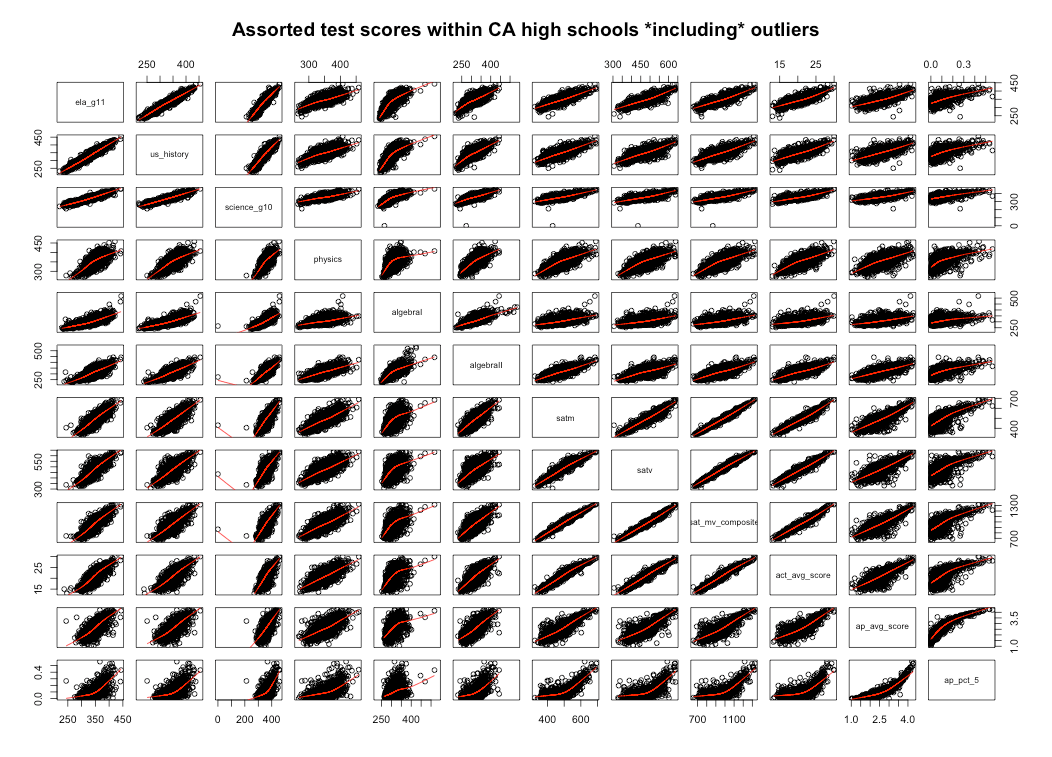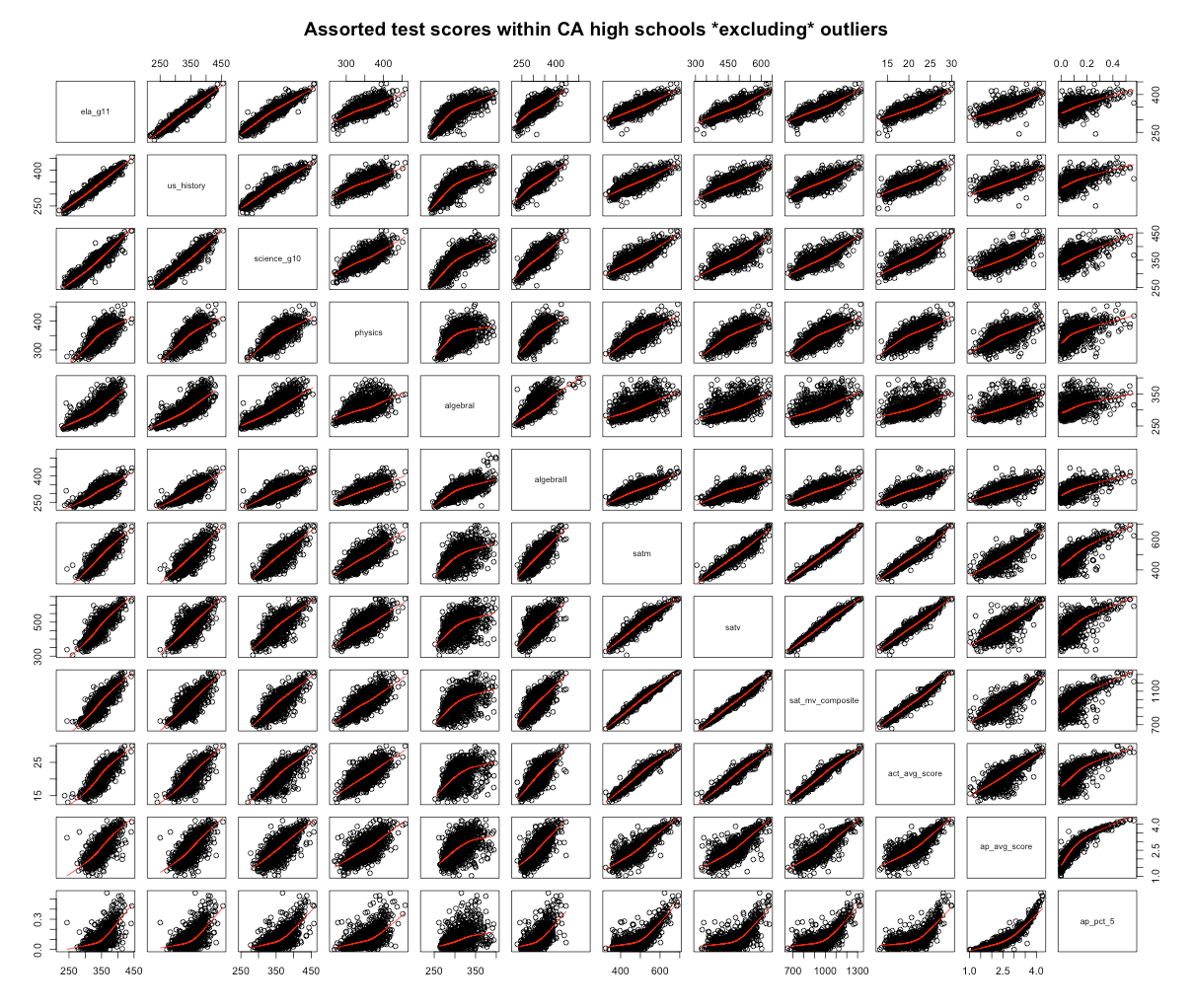To exploit some of my prior work with California’s test score data, I decided to extend this analysis to SAT, ACT, and AP scores in the state of California, i.e., to compare the relationships of these different tests within and between schools.

Notes/Caveats:

• To achieve more stable results with schools with small numbers of test takers I averaged 2-4 years worth of test score data together.
• Unfortunately, AP results are not broken out by subject (some are much harder than others and there are apt to be different test taking patterns at different sorts of schools)
• SAT/AP/ACT data is not available by race/ethnicity

ACT vs SAT (r=0.97)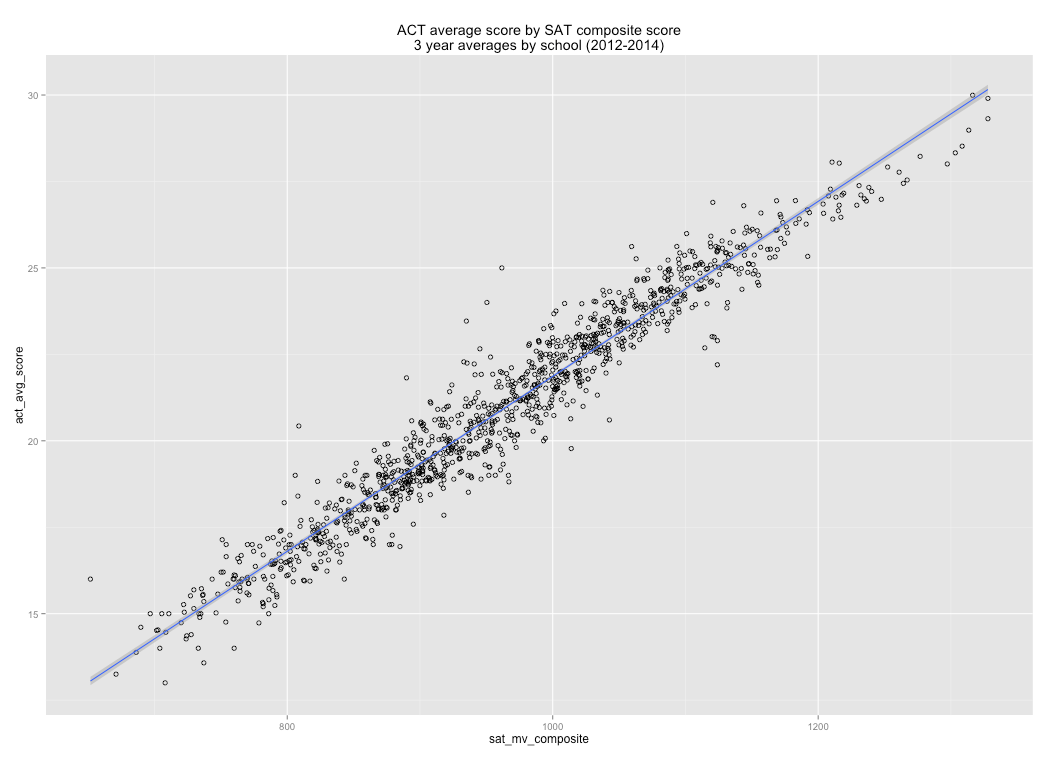[It’s almost like they’re testing the same construct…. :-)]

SAT by English grade 11 (r=0.84)SAT by Science Grade 10 (r=0.81)SAT by World History (r=0.79)SAT by Physics (r=0.73)SAT by Algebra I (r=0.56)SAT math by Algebra I (r=0.57)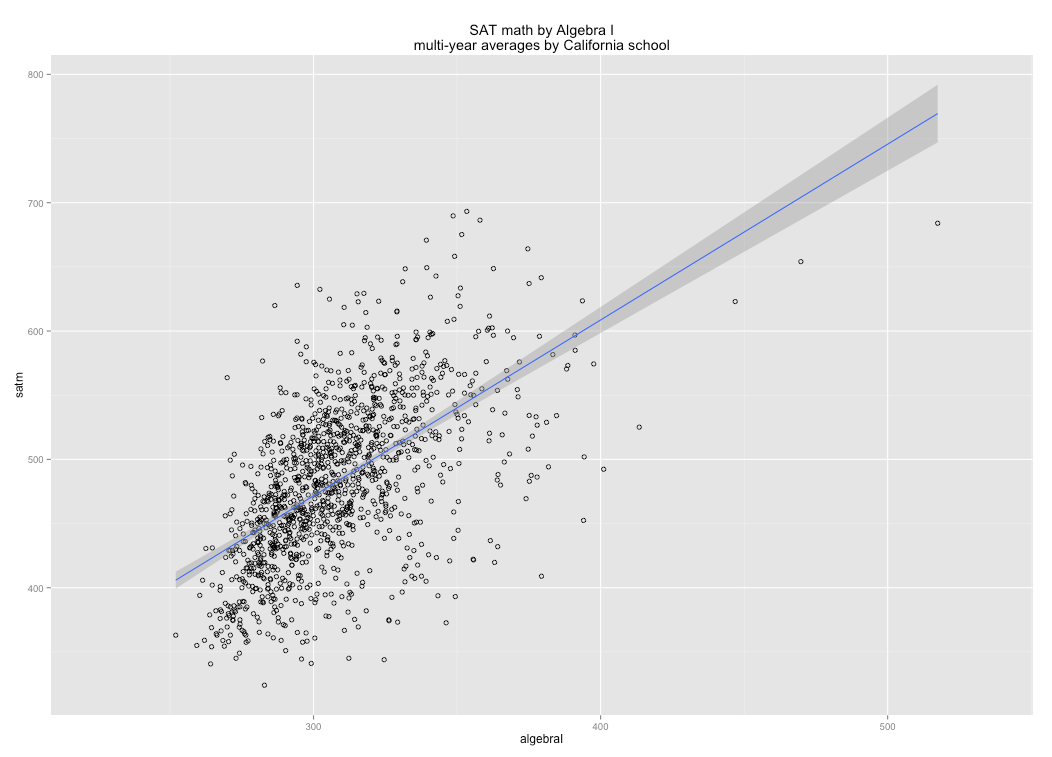SAT by Algebra II (r=0.75)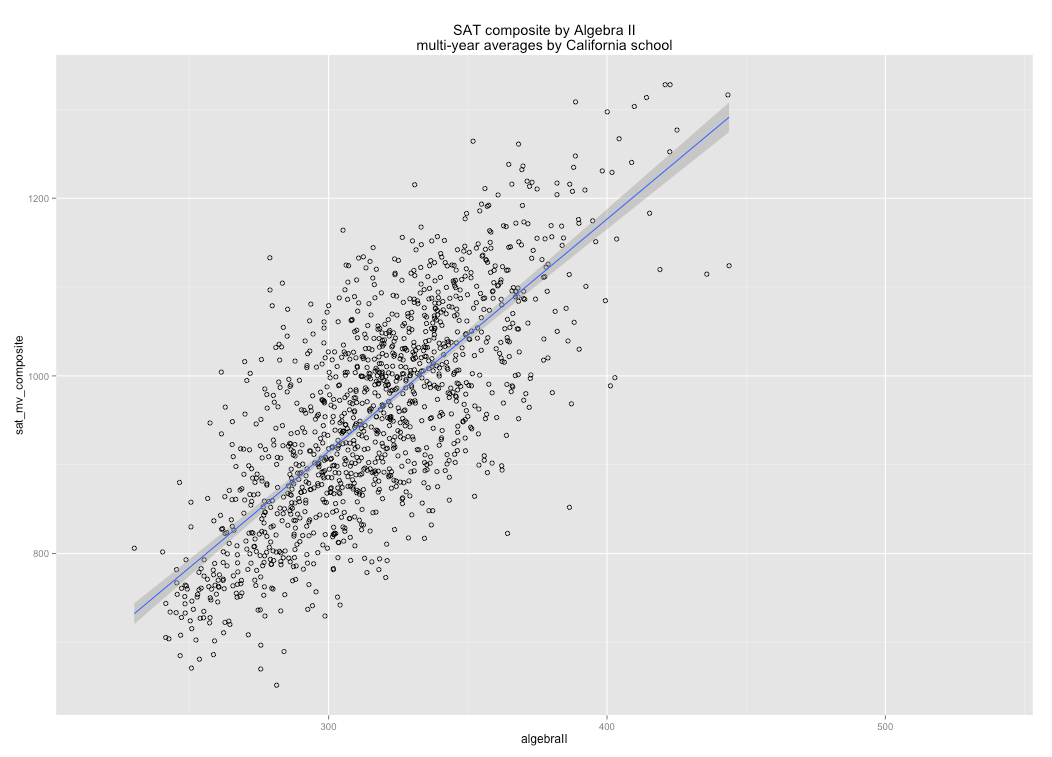SAT math by Algebra II (r=0.79)AP Average score by Algebra II (r=0.70)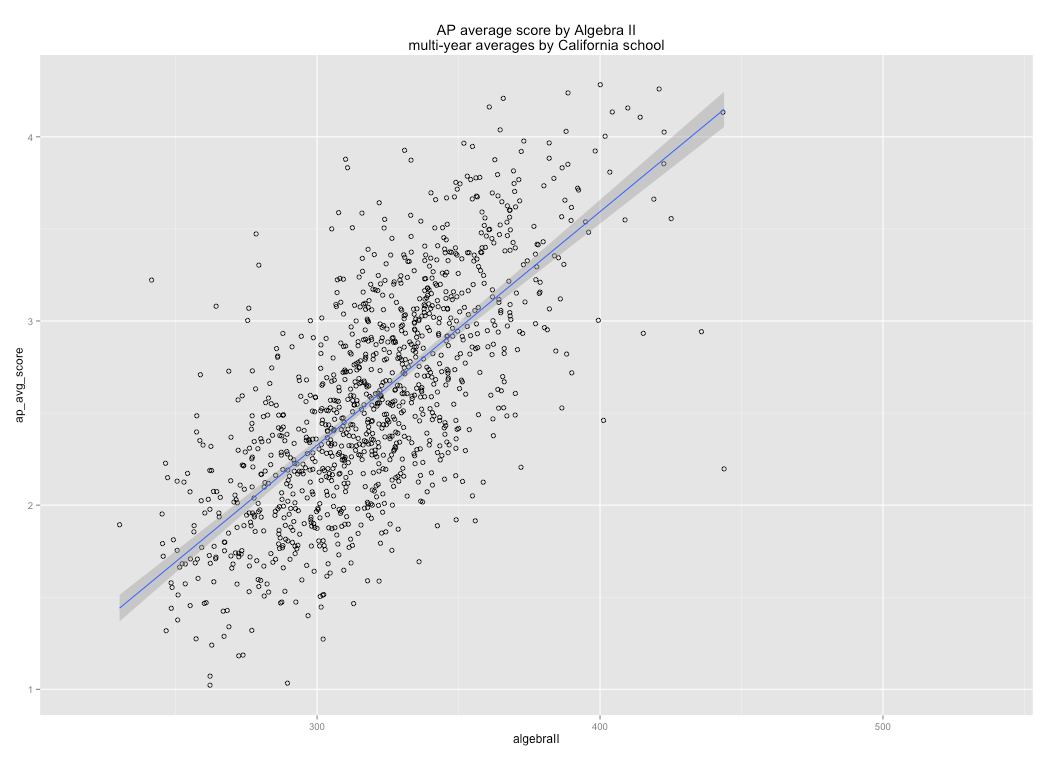AP average score by English Grade 11 (r=0.72)AP average score by Science Grade 10 (r=0.70)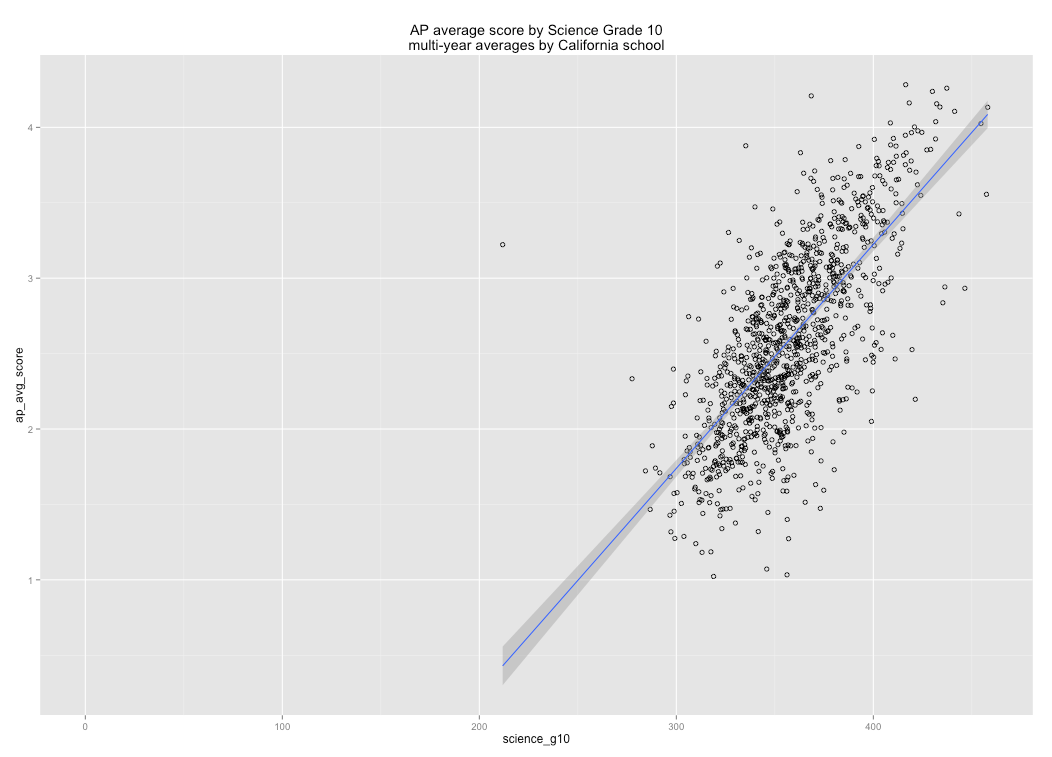AP by SAT composite (r=0.82)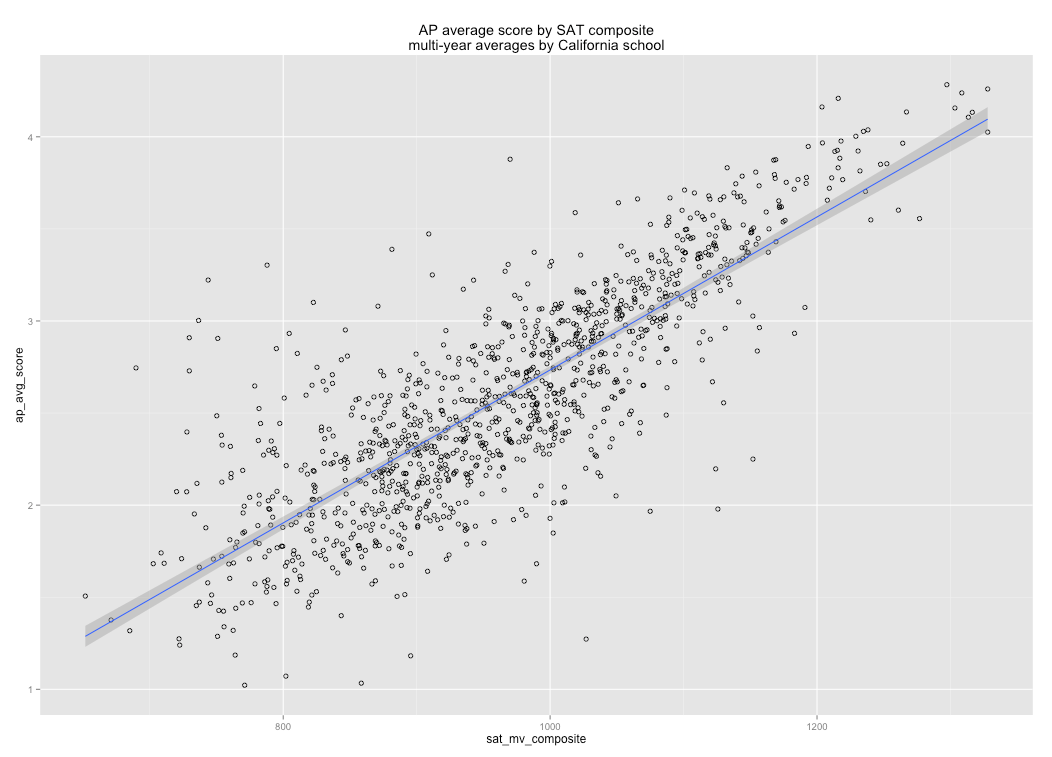AP by ACT average score (r=0.81)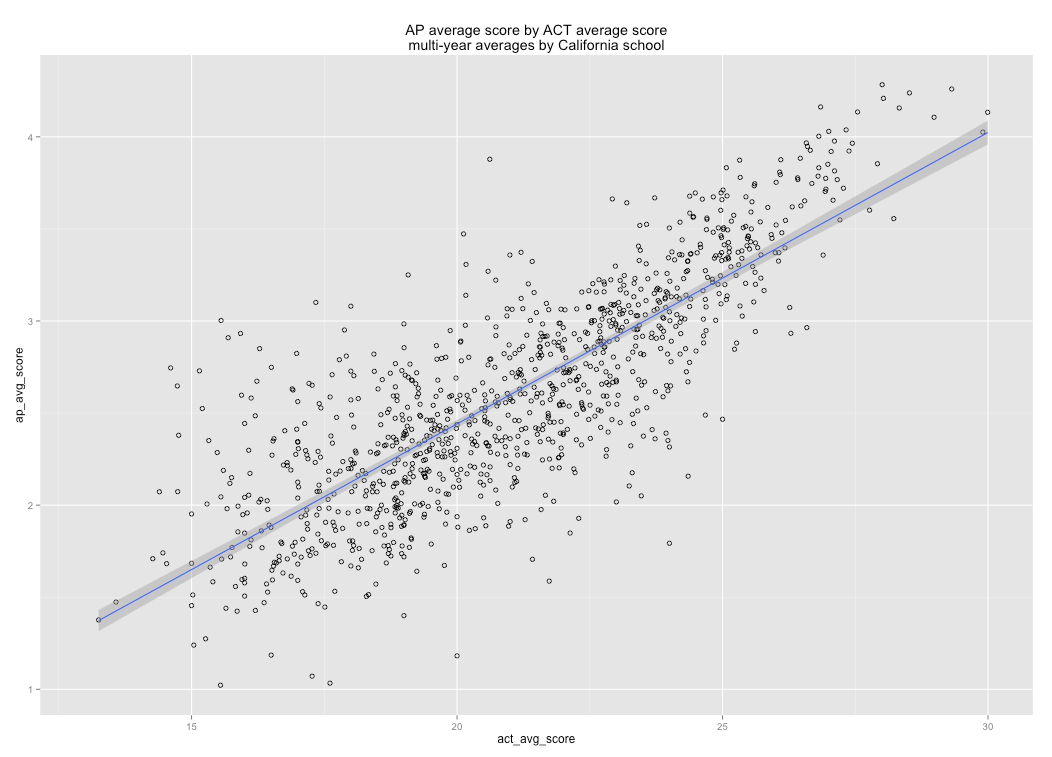AP percent scoring 5 by ACTAP percent scoring 4 or higher by ACT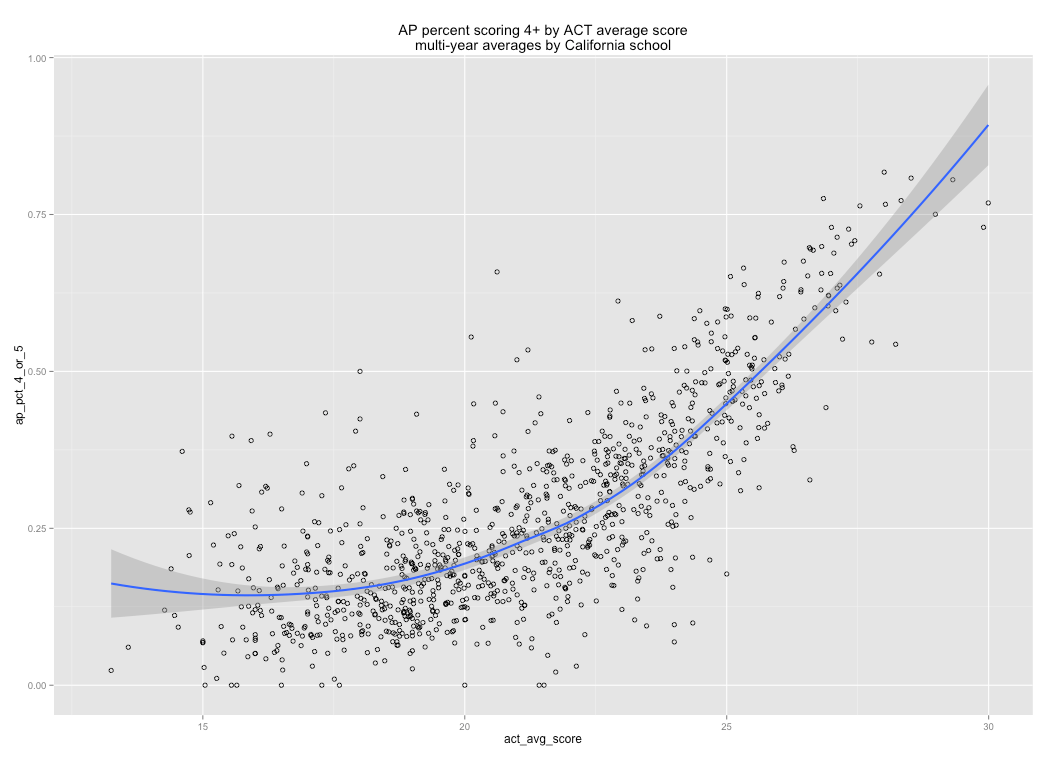AP percent scoring 3 or higher by ACTAP percent scoring 1 by ACT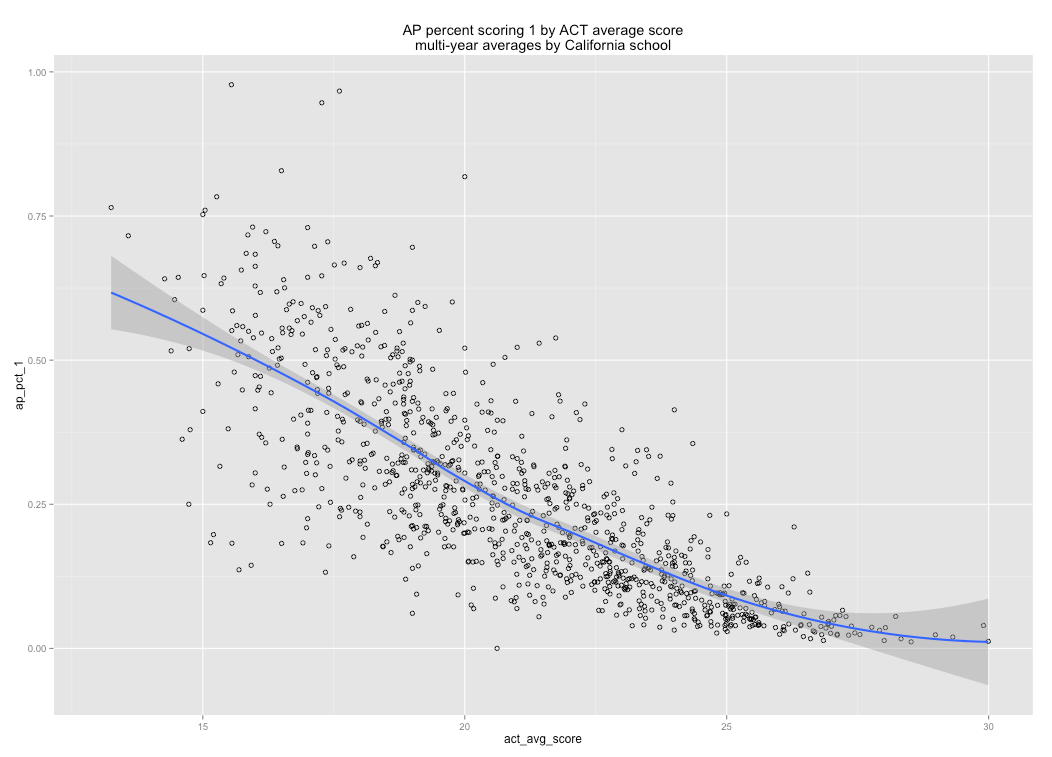AP Percent scoring 2 or lowerOther correlates

Average number of AP tests per test taker by (school) ACT score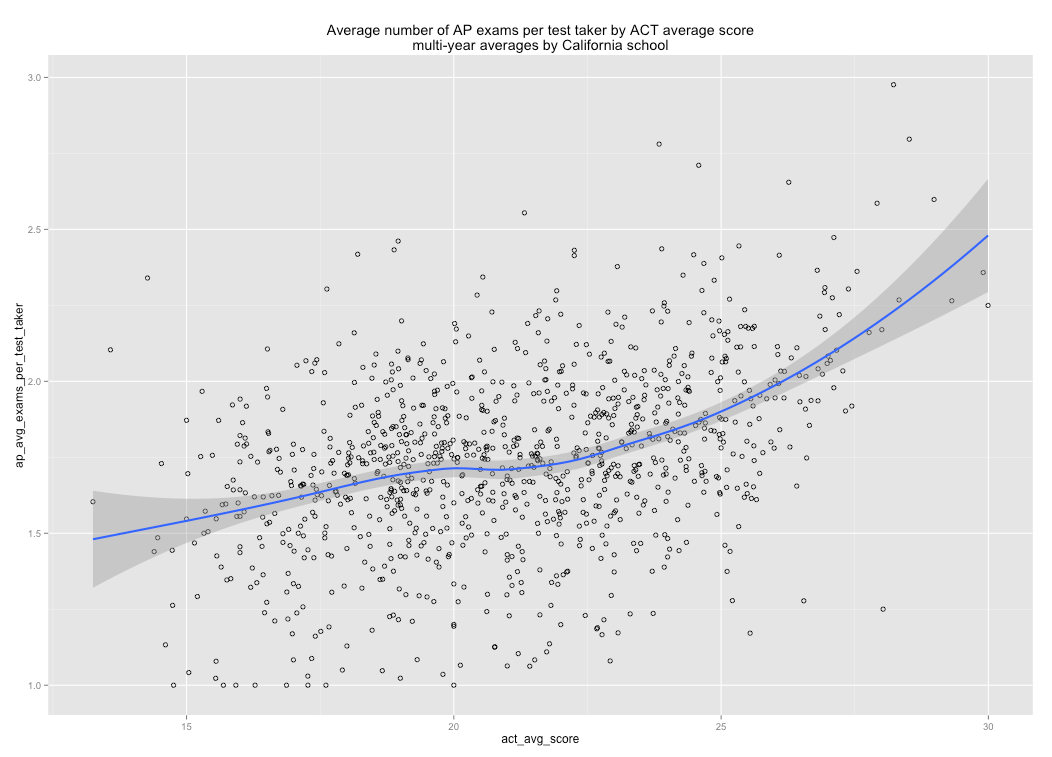Average number of tests per senior by ACT score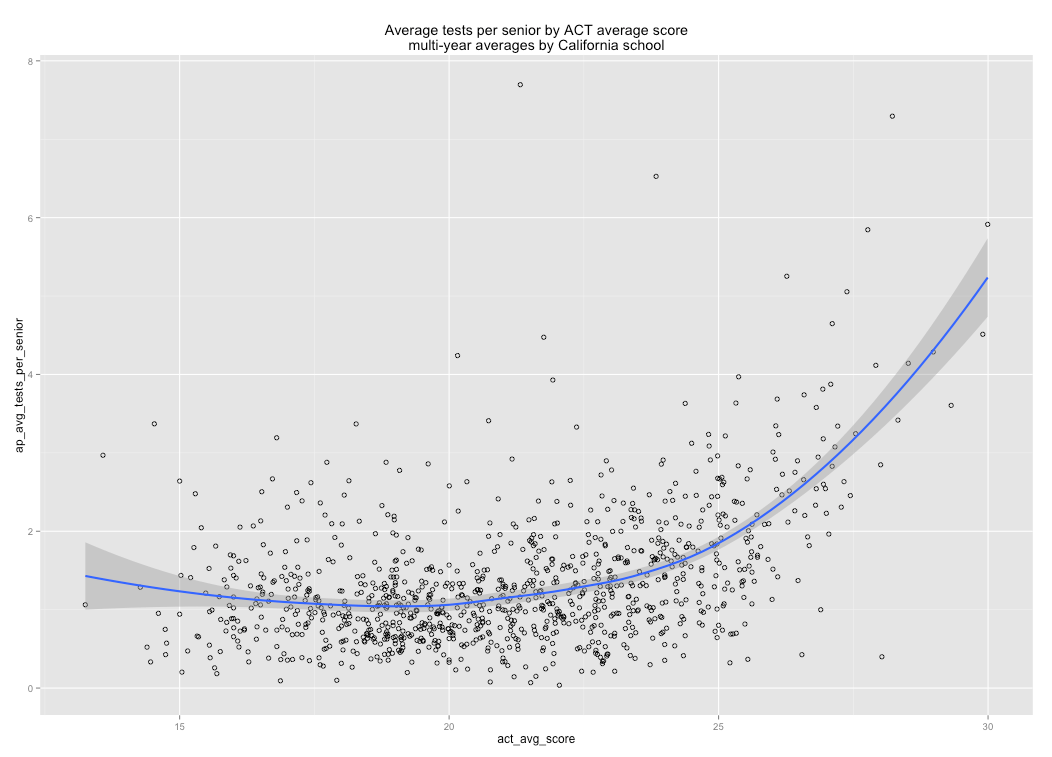Average percent of senior tests by ACT score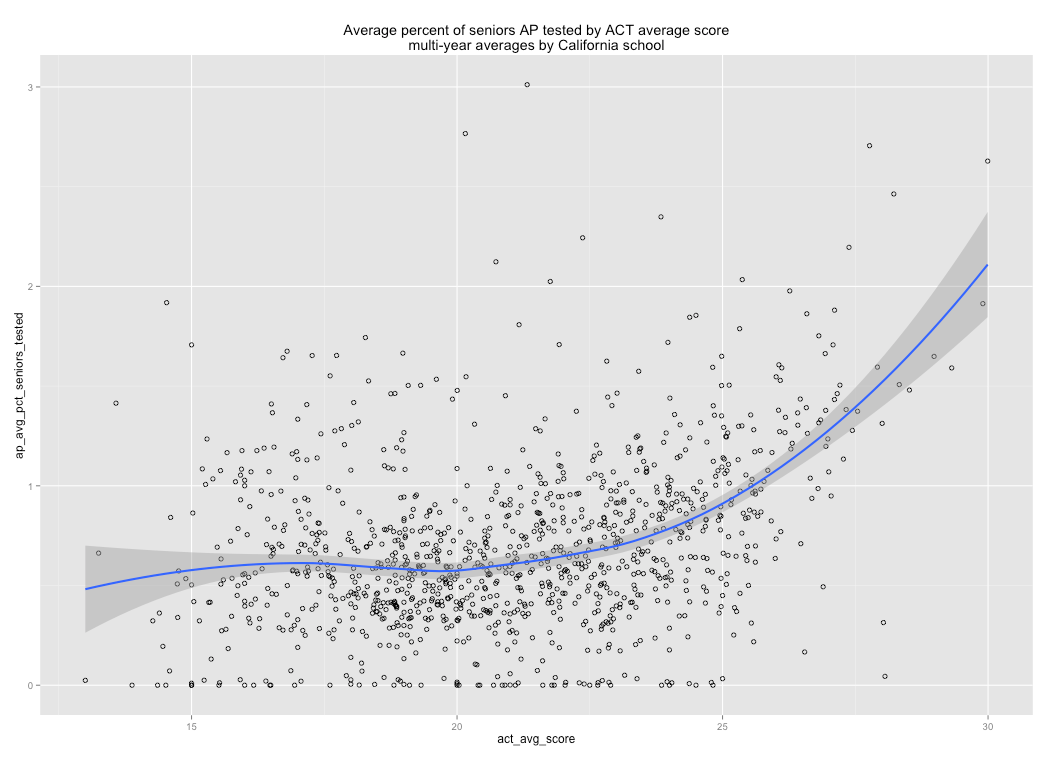Note: I am simply dividing by the number of students enrolled in grade 12.  Since juniors can and do take these tests the totals can exceed 100%.

Percent of seniors taking SAT (test takers / # seniors) by English Grade 11 scoresPercent of seniors taking ACT tests (test takers / # seniors) by English Grade 11 scores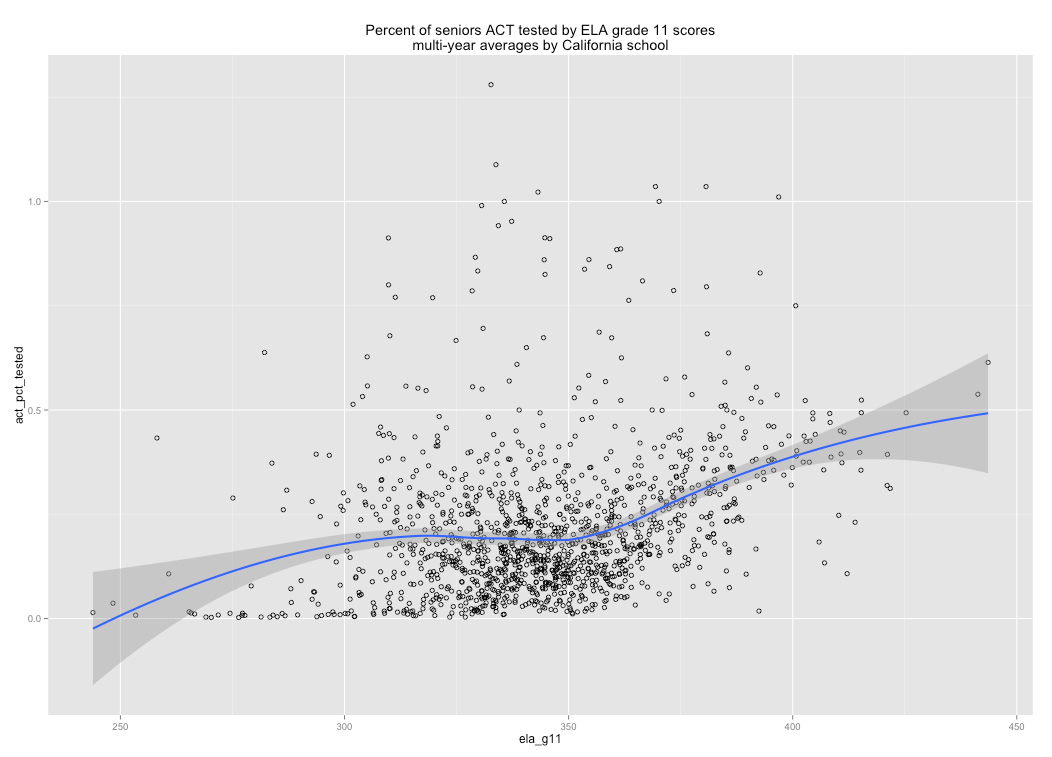Percent of seniors taking SAT by ACT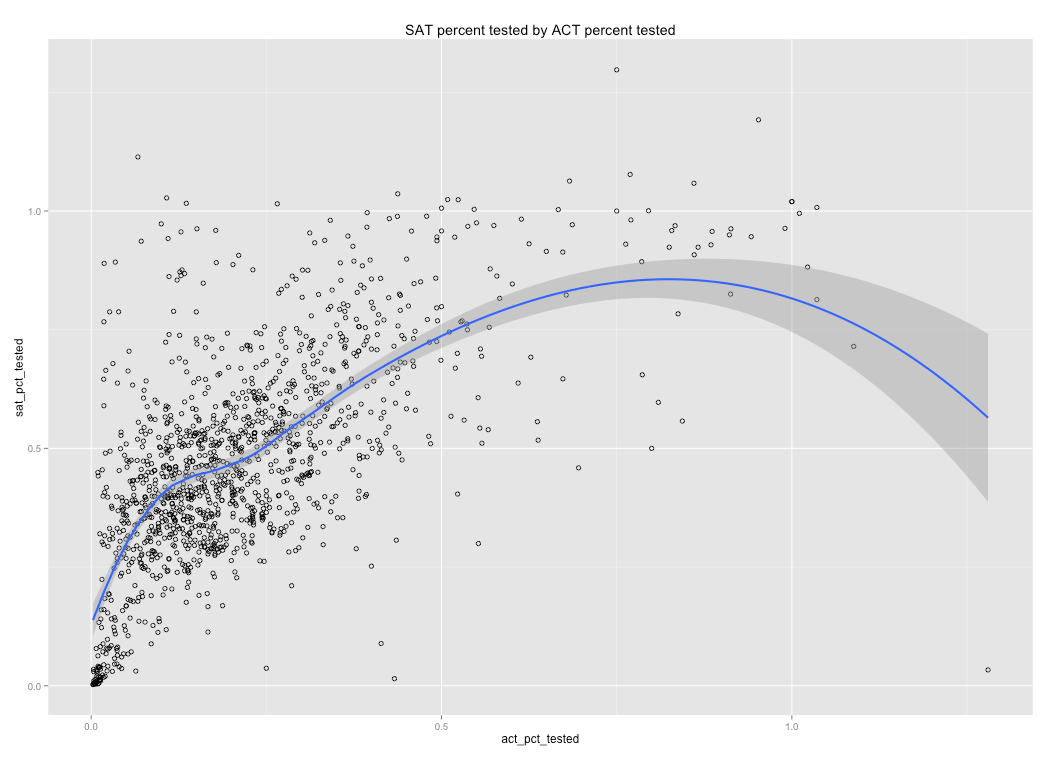AP percent tested by SAT percent tested (test takers / reported senior enrollment)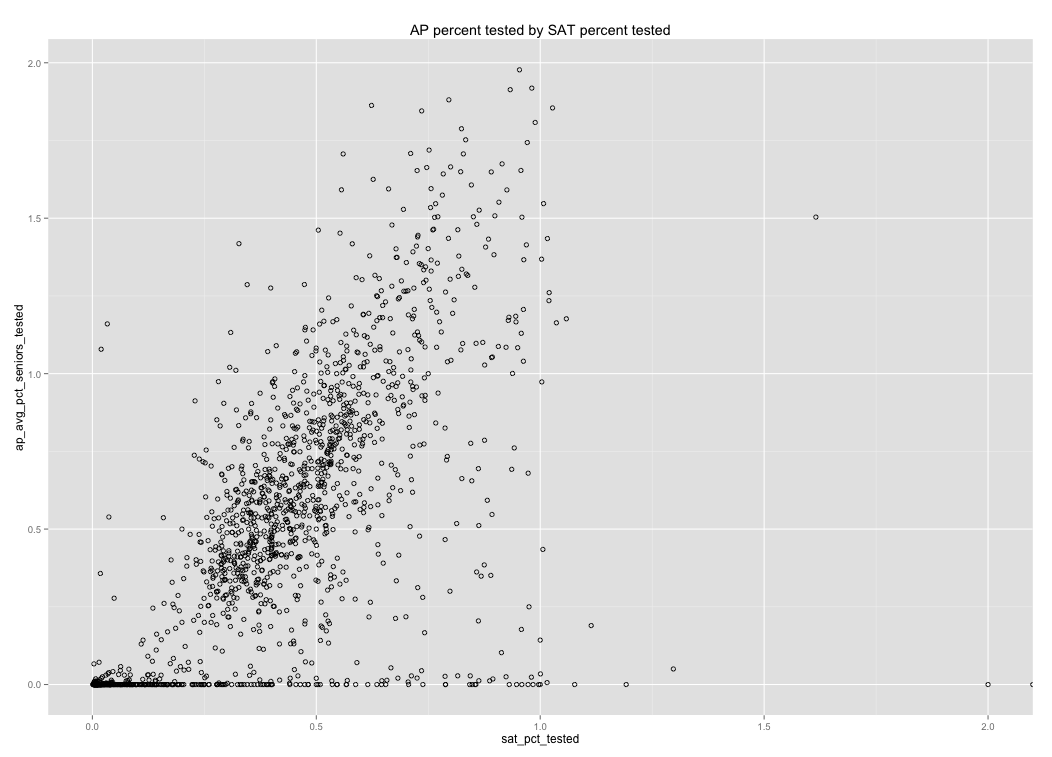SAT composite by percent of “seniors” taking SAT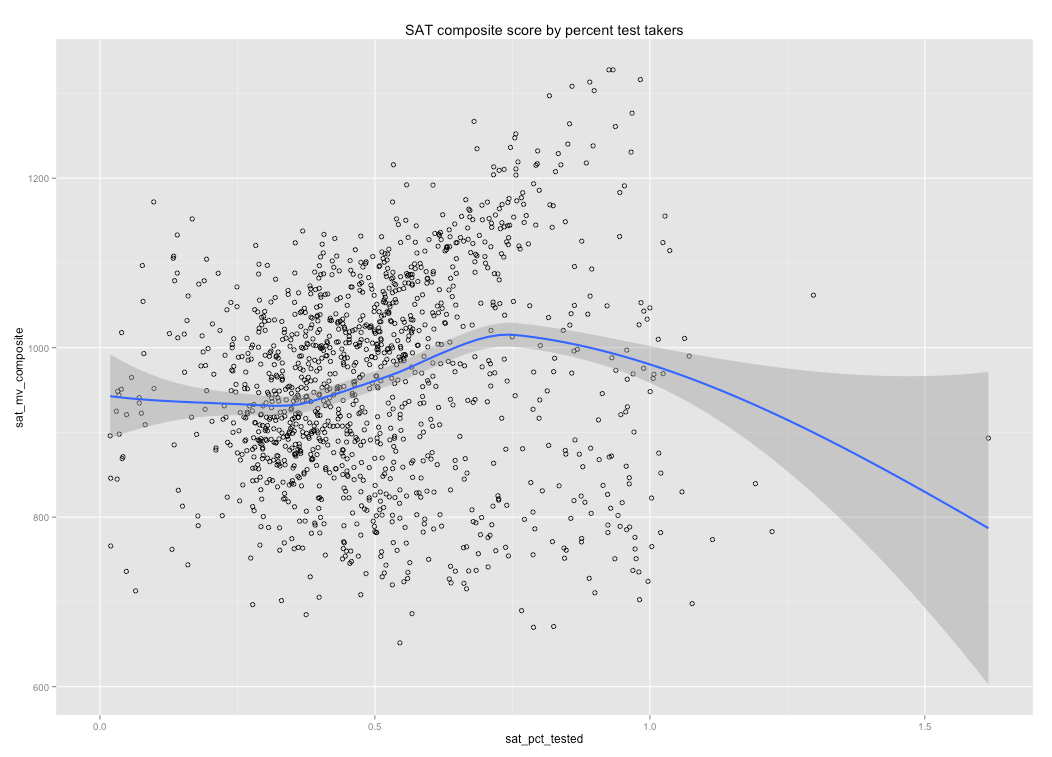Some quick and dirty modeling exercises

Model 1 (ELA grade 12 + percent seniors tested): r=0.88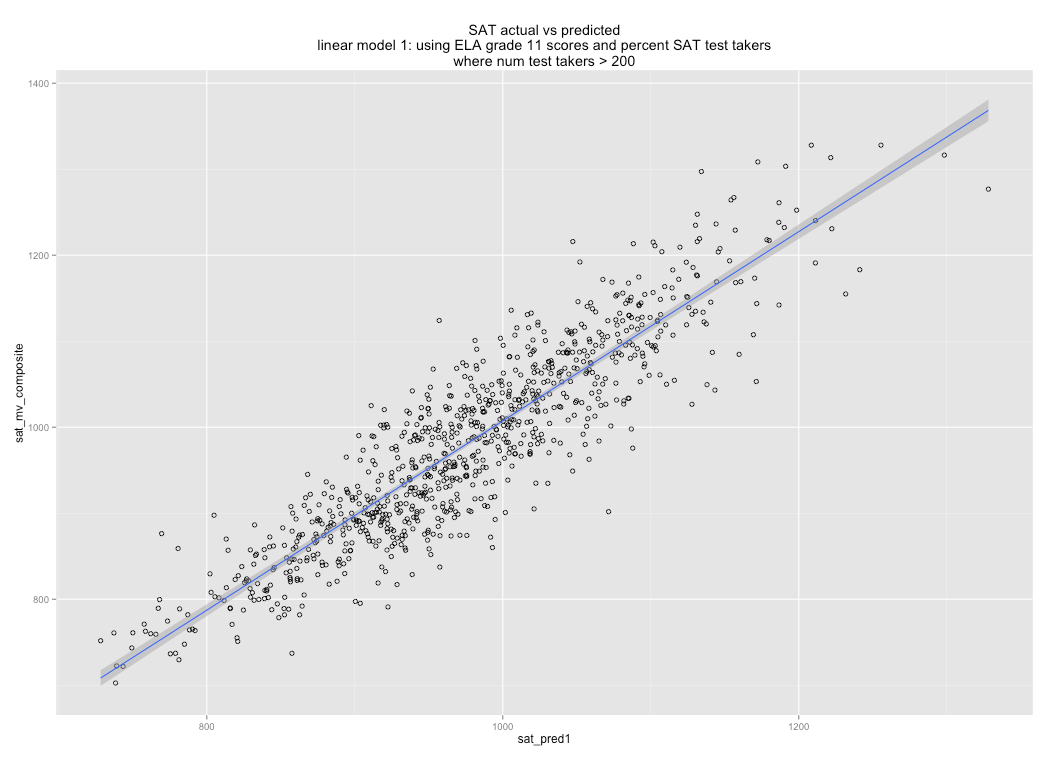Model 2 (Grade 10 Science + Algebra II + percent SAT test takers), r=0.85I’m sure I could do better than this (especially if I deal with some of those outliers).  My point here is simply this: these tests are highly correlated and that accounting for differences in test taking rates or aggregating a few of them together improves the relationships further.

Scatterplot matrices